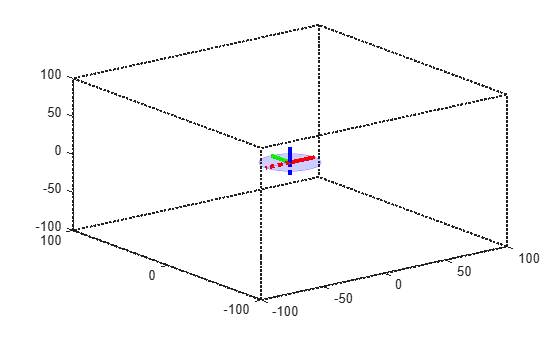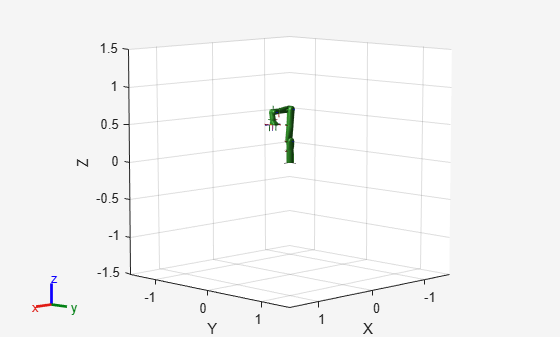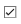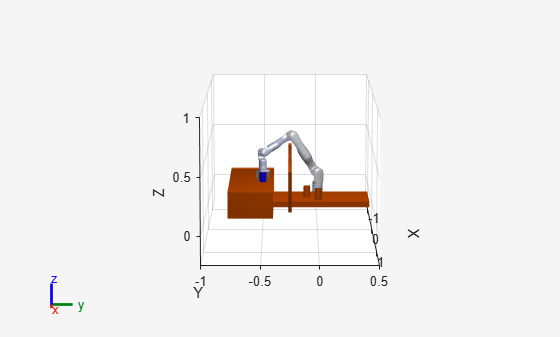# Plan Paths With End-Effector Constraints Using State Spaces For Manipulators

Plan a manipulator robot path using sampling-based planners like the rapidly-exploring random trees (RRT) algorithm.

This example uses the `manipulatorStateSpace` and `manipulatorCollisionBodyValidator` objects as a state space and state validator that works with sampling-based planners, like the `plannerBiRRT` object available with Navigation Toolbox™. In this example, you define the custom behavior of a manipulator state space to ensure end-effector constraints are met. You plan a path to pick and place a container with a constrained end effector that must remain upright throughout the path. This constraint could be for a container of fluids, a welding tool path, or drilling application where you must keep the end-effector pose in a fixed orientation.

### Define State Space

To create a constrained state space, generate a class `exampleHelperConstrainedStateSpace` that derives from `manipulatorStateSpace`. Open this example to get the supporting file.

```classdef exampleHelperConstrainedStateSpace < manipulatorStateSpace ```

Specify the constructor syntax which requires the robot model, end effector name, and target orientation as inputs. Define a property called `EnableConstraint` for turning the constraint on and off.

The constrained region is defined in the constructor using a `workspaceGoalRegion` object. The bounds on the xyz-position are all `[-100,100]` meters. The orientation bounds are constrainted to any rotation `[-pi pi]` about the z-axis with zero rotation in x and y.

Create an inverse kinematics (IK) solver, and specify any solver parameters. This solver generates the joint configurations of the robot for the end-effector poses sampled inside the workspace.

``` function obj = exampleHelperConstrainedStateSpace(rbt,endEffectorName,targetOrientation) %Constructor obj@manipulatorStateSpace(rbt); obj.EnableConstraint = true; obj.Region = workspaceGoalRegion(endEffectorName,'EndEffectorOffsetPose',targetOrientation); obj.Region.Bounds = [-100 100; -100 100; -100 100; -pi pi; 0 0; 0 0]; % Store a reference of the manipulatorStateSpace/RigidBodyTree % in the Robot property. Note that the RigidBodyTree property % of the manipulatorStateSpace is read-only. Hence, accessing % it will involve creating a copy of the underlying handle, % which can be expensive. obj.Robot = obj.RigidBodyTree; % Configure the IK solver obj.IKSolver = inverseKinematics('RigidBodyTree', obj.Robot); obj.IKSolver.SolverParameters.AllowRandomRestart = false; end ```

### Customize Interpolation Method

During the planning phase, the `interpolate` function connects configurations in the search tree. By default, the interpolation is unconstrained. Customize the `interpolate` function to ensure that the end-effector remains upright. Override the `interpolate` method on the `manipulatorStateSpace` object with this code.

``` function constrainedStates = interpolate(obj, state1, state2, ratios) constrainedStates = interpolate@manipulatorStateSpace(obj,state1,state2,ratios); if(obj.EnableConstraint) for i = 1:size(constrainedStates, 1) constrainedStates(i,:) = constrainConfig(obj,constrainedStates(i,:)); end end end ```

The `constraintConfig` function replaces the unconstrained joint configurations with constrained configurations. It finds the end-effector pose closest to the constrained region  and returns the corresponding robot joint configuration. Given the end-effector pose ${\mathit{T}}_{\mathit{s}}^{0}$ corresponding to `config`, the function computes the pose closest to the end-effector pose in the constrained region and returns the corresponding robot joint configuration.

To find the closest pose in the reference frame, first find the displacement of ${\mathit{T}}_{\mathit{s}}^{0}$ from the region by calculating ${\mathit{T}}_{{\mathit{s}}^{\prime }}^{\mathit{w}}={{\mathit{T}}_{\mathit{w}}^{0}}^{-1}{\mathit{T}}_{\mathit{s}}^{0}\text{\hspace{0.17em}}{{\mathit{T}}_{\mathit{e}}^{\mathit{w}}}^{-1}$ and converting ${\mathit{T}}_{{\mathit{s}}^{\prime }}^{\mathit{w}}$ to a pose vector close to the bounds of the region. Given the bounds, there are three possibililties for an element in the pose vector and its corresponding bounds of the region:

1. The value is within bounds. In this case the element remains unchanged as the pose element is within the region.

2. The value is greater than the maximum value of the bound. In this case, clip it to the max value of the bound.

3. The value is lesser than the min value of the bound. In this case, clip it to the min value of the bound.

Once the resulting closest constrained pose is found in the reference frame, convert this to a pose in the world coordinates.

``` function constrainedConfig = constrainConfig(obj, config) %constrainConfig Constraint joint configuration to the region % The function finds the joint configuration corresponding to % the end-effector pose closest to the constrained region. wgr = obj.Region; T0_s = obj.Robot.getTransform(config, wgr.EndEffectorName); T0_w = wgr.ReferencePose; Tw_e = wgr.EndEffectorOffsetPose; Tw_sPrime = T0_w \ T0_s / Tw_e; dw = convertTransformToPoseVector(obj, Tw_sPrime); bounds = wgr.Bounds; % Find the pose vector closeset to the bounds of the region. for dofIdx = 1:6 if(dw(dofIdx) < bounds(dofIdx, 1)) dw(dofIdx) = bounds(dofIdx, 1); elseif(dw(dofIdx) > bounds(dofIdx, 2)) dw(dofIdx) = bounds(dofIdx, 2); end end % Convert the pose vector in the region's reference frame to a % homogeneous transform. constrainedPose = obj.convertPoseVectorToTransform(dw); % Convert this pose to world coordinates, and find the % corresponding joint configuration. constrainedPose = T0_w * constrainedPose * Tw_e; constrainedConfig = obj.IKSolver(obj.Region.EndEffectorName, ... constrainedPose, ... ones(1, 6), ... config); end ```

### Create Robot State Space

Now that you have setup your constrained state space, load an example robot and environment using the `exampleHelperEndEffectorConstraintedEnvironment` function. The output `kinova` is a rigid body tree robot model of the KINOVA™ Gen 3 and `env` is a cell array of collision body objects in the robot world.

`[kinova,env] = exampleHelperEndEffectorConstrainedEnvironment;`

Specify the end-effector name and target orientation. Store the joint position values for opening and closing the gripper.

```endEffectorName = "EndEffector_Link"; targetOrientation = eul2tform([0 pi 0]); openPostion = 0.06; closedPosition = 0.04;```

Create the customized state space.

```% prevSeed = rng rng default ss = exampleHelperConstrainedStateSpace(kinova, endEffectorName, targetOrientation); % currSeed = rng % assert(all(prevSeed.State == currSeed.State))```

Specify state bounds on the gripper position so it remains open.

`ss.StateBounds(end-1:end,:) = repmat([openPostion, openPostion], 2, 1);`

Visualize the constrained `workspaceGoalRegion` region.

```figure; show(ss.Region)```### Create State Validator

Create a `manipulatorCollisionBodyValidator` object from the state space and add the environment of collision objects. To improve performance, ignore self collisions when valdiating the state space. The robot model uses joint limits to ensure that self collisions will not occur.

```sv = manipulatorCollisionBodyValidator(ss,"Environment",env); sv.IgnoreSelfCollision = true;```

### Create Planner

This example uses the `plannerBiRRT` object, which is a bi-directional variant of the RRT algorithm with the "connect" heuristic enabled. For a sparse environment, this planner finds a solution in lesser number of iterations as compared to other RRT-based planners . Alternatively, for shorter paths with trimmed edges, consider the `plannerRRTStar` object.

The robot starts in a joint configuration which satisfies the constraint. Alternatively, the `interpolate` function of the constrained state space can be used to constrain the start configuration. This ensures that all the states in the resulting plan are constrained. Set the random number seed for repeatable results.

```rng(20); planner = plannerBiRRT(ss, sv); planner.MaxConnectionDistance = 0.5; planner.EnableConnectHeuristic = true; startConfig = [0.1196 -0.045 -0.1303 1.6528 -0.0019 1.5032 0.0083, openPostion, openPostion]; figure("Visible","on"); show(kinova, startConfig, ... "Visuals", "off", ... "Collisions", "on", ... "PreservePlot", true);```### Define Grasp Region

Next, define a grasping region using the `workspaceGoalRegion` object which represents a cylinder to pick in the environment (`env{3}`). Generate a joint configuration for a sampled end-effector pose. You should ensure that the configuration passed to the planner is collision-free.

```graspingRegion = workspaceGoalRegion(endEffectorName); % Attach the reference frame to the pose of the cylinder object. graspingRegion.ReferencePose = env{3}.Pose; % Define the offset of the end-effector relative to the target orientation. graspingRegion.EndEffectorOffsetPose = trvec2tform([0, 0, 0.07]) * targetOrientation; % Generate goal joint configuration of the robot given a sampled end-effector pose in % the region. goalConfig = jointConfigurationGiven(ss,sample(graspingRegion)); goalConfig(end-1:end) = openPostion;```

### Plan Path

Call the `plan` function to plan a path between the start and goal configurations. Time the planning phase. Interpolate the path to 50 points.

```tic; pickPath = plan(planner,startConfig,goalConfig);```
```ans = 0.0536 ```
```ans = 0.6800 ```
```ans = 0.9189 ```
```tOut = toc; interpolate(pickPath,50);```
```ans = 0.7108 ```
```ans = 0.3126 ```
```fprintf(newline); fprintf("Planning finished in %d s\n", tOut);```
```Planning finished in 4.554262e+00 s ```

Visualize the planned path. The robot moves to the container to grab it.

```hold on; ylim([-1 0.5]) zlim([-0.25 1]) for i = 1:length(env) show(env{i}) end for i = 1:size(pickPath.States, 1) show(kinova, pickPath.States(i, :), ... "Visuals", "on", ... "FastUpdate",true, ... "Frames", "off", ... "PreservePlot",false); drawnow; end exampleHelperConstrainedRobotMoveGripper(kinova,... pickPath.States(end,:),openPostion,closedPosition); hold off;```### Recreate Planner

Attach the collision geometry of the can to the end-effector and remove the can from the environment. Then, with the modified robot, create a planning instance with the new robot model. After planning, you could unceck `ss.EnableContraint` and rerun this script to see a path without a constrainted end effector.

```zOffset = [0 0 (env{3}.Length)/2]; container = env{3}; addCollision(kinova.getBody(endEffectorName),env{3}, (env{3}.Pose) \ trvec2tform(zOffset)); env(3) = []; ss = exampleHelperConstrainedStateSpace(kinova,endEffectorName,targetOrientation); % Keep the end-effector closed during planning ss.StateBounds(end-1:end,:) = repmat([0.04 0.04],2,1); sv = manipulatorCollisionBodyValidator(ss, ... "Environment",env,"ValidationDistance",0.2); planner = plannerBiRRT(ss,sv); planner.MaxConnectionDistance = 1; planner.EnableConnectHeuristic = true; ss.EnableConstraint =true;```

### Plan Path

Next, plan from the last configuration in the previous path to a new goal joint configuration. The goal configuration places the can on the opposite table in the environment.

```% The last state of the of the previous plan startConfig = pickPath.States(end,:); startConfig(end-1:end) = 0.04; goalConfig = [1.1998 0.6044 0.0091 1.4219 -0.0058 1.1154 0.0076 0.04 0.04]; rng(10); tic placePath = plan(planner,startConfig,goalConfig);```
```ans = 0.0661 ```
```ans = 0.9539 ```
```ans = 0.8572 ```
```ans = 0.2097 ```
```ans = 0.4645 ```
```ans = 0.6127 ```
```ans = 0.0560 ```
```ans = 0.2713 ```
```tOut = toc; fprintf("Planning finished in %0.2d s\n",tOut);```
```Planning finished in 5.17e+00 s ```
`interpolate(placePath,50);`
```ans = 0.6251 ```
```ans = 0.7755 ```
```ans = 0.3552 ```

Visualize the path that places the container. Notice that the can remains upright throughout the path.

```show(kinova, startConfig, ... "FastUpdate", false, ... "PreservePlot", false); view([90,0,15]) ylim([-1 0.5]) zlim([-0.25 1]) hold on; for i = 1:length(env) show(env{i}) end containerPose = hgtransform(); containerPose.Matrix = container.Pose; [ax, p] = show(container); p.FaceColor = [0 0 1]; p.Parent = containerPose; for i = 1:size(placePath.States, 1) cPose = getTransform(kinova,placePath.States(i, :),endEffectorName); containerPose.Matrix = cPose/(container.Pose)*trvec2tform(zOffset); show(kinova,placePath.States(i,:), ... "FastUpdate",true, ... "PreservePlot",false, ... "Frames", "off"); drawnow; end exampleHelperConstrainedRobotMoveGripper(kinova,... placePath.States(end,:),closedPosition,openPostion); hold off;```### Next Steps

To see an unconstrained path, uncheck the `ss.EnableConstraint` checkbox and rerun the script. The cup tilts in a way that could easily spill the contents. You could also consider replacing the `plannerBiRRT` object with another sampling-based planner like `plannerRRTStar` to evaluate their performance.

### References

 D. Berenson, S. Srinivasa, D. Ferguson, A. Collet, and J. Kuffner, "Manipulation Planning with Workspace Goal Regions", in Proceedings of IEEE International Conference on Robotics and Automation, 2009, pp.1397-1403